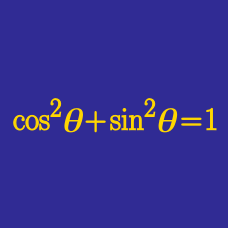Geometry

# Trigonometric R method

Which of the following is equal to $4\sin x+4\cos x?$

As $\theta$ ranges over all real values, what is the maximum value of

$(18 \sin \theta + 3 \cos \theta ) ^2 ?$

What are the maximum and minimum values of $-16\sin x+16\sqrt{3}\cos x?$

In the interval $[0,4\pi],$ find the sum of all the solutions to the equation $2\sqrt{6}\sin\left(x+\frac{\pi}{5}\right)-2\sqrt{2}\cos\left(x+\frac{\pi}{5}\right)=2\sqrt{6}.$

What is the largest possible value of $15\sin x +8\cos x,$ where $x$ is a real number?

×